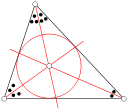# Definition of Incircle of TriangleAn incircle of a triangle is the largest circle that will fit inside a triangle, just touching each of the triangle's sides.

Another term for the incircle of a triangle is the inscribed circle.

The centre of the incircle of a triangle is called its "incentre". It is the place where the bisectors the three angles of the triangle intersect.

### Description

The aim of this dictionary is to provide definitions to common mathematical terms. Students learn a new math skill every week at school, sometimes just before they start a new skill, if they want to look at what a specific term means, this is where this dictionary will become handy and a go-to guide for a student.

### Audience

Year 1 to Year 12 students

### Learning Objectives

Learn common math terms starting with letter I

Author: Subject Coach
You must be logged in as Student to ask a Question.